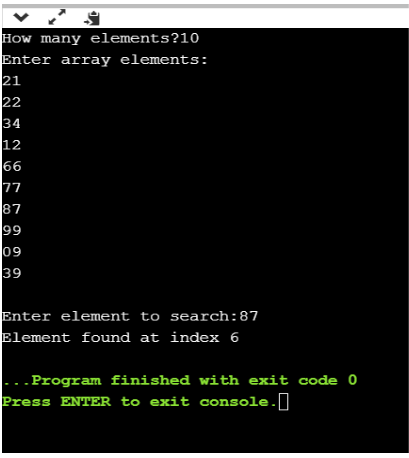# How to Implement Linear Search in C?

Last updated on Sep 19,2019 3.3K ViewsHow to Implement Linear Search in C?

Linear search is a very simple and basic search algorithm. In this blog on “Linear search in C”, we will implement a C Program that finds the position of an element in an array using a Linear Search Algorithm.

We will be covering the following topics in this blog:

## What is a Linear Search?

A linear search, also known as a sequential search, is a method of finding an element within a list. It checks each element of the list sequentially until a match is found or the whole list has been searched.

## A simple approach to implement a linear search is

• Begin with the leftmost element of arr[] and one by one compare x with each element.

• If x matches with an element then return the index.

• If x does not match with any of the elements then return -1.

## Implementing Linear Search in C

```#include<stdio.h>

int main()
{
int a,i,x,n;
printf("How many elements?");
scanf("%d",&n);

printf("Enter array elements:n");
for(i=0;i<n;++i)
scanf("%d",&a[i]);

printf("nEnter element to search:");
scanf("%d",&x);

for(i=0;i<n;++i)
if(a[i]==x)
break;

if(i<n)
printf("Element found at index %d",i);
else

return 0;
}
```

Output:The time required to search an element using a linear search algorithm depends on the size of the list. In the best-case scenario, the element is present at the beginning of the list and in the worst-case, it is present at the end.

The time complexity of a linear search is O(n).

With this, we come to the end of this blog on ‘Linear Search in C’. I hope you found it informative.

Now that you have understood the basics of Programming in C, check out the training provided by Edureka on many technologies like Java, Spring and  many more, a trusted online learning company with a network of more than 250,000 satisfied learners spread across the globe

Got a question for us? Mention it in the comments section of this “Linear Search in C” blog and we will get back to you as soon as possible.